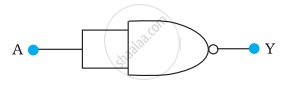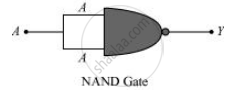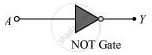# Write the truth table for a NAND gate connected as given in following figure. Hence identify the exact logic operation carried out by this circuit - Physics

Numerical

Write the truth table for a NAND gate connected as given in the following figure.Hence identify the exact logic operation carried out by this circuit

#### Solution

A acts as the two inputs of the NAND gate and Y is the output, as shown in the following figure.Hence, the output can be written as:

Y =  bar("A"."A") = bar"A" + bar"A" = bar"A"    ...(i)

The truth table for equation (i) can be drawn as:

 A Y(=bar"A") 0 1 1 0

This circuit functions as a NOT gate. The symbol for this logic circuit is shown as:Concept: Digital Electronics and Logic Gates
Is there an error in this question or solution?

#### APPEARS IN

NCERT Physics Part 1 and 2 Class 12
Chapter 14 Semiconductor Electronics: Materials, Devices and Simple Circuits
Exercise | Q 14.12 | Page 498
NCERT Class 12 Physics Textbook
Chapter 14 Semiconductor Electronics: Materials, Devices and Simple Circuits
Exercise | Q 16 | Page 511

Share